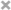# Xiuchuan Zhang

Personal Website

This is Xiuchuan's personal website.
I plan to post some of my current learning and review notes on it.
If you have any questions or suggestions, welcome to comment in my posts.

This review is for myself further learning to find notations def. & thm. easier
Source of the notes:
Wikipedia
Linear Analysis Lecture Notes
Boundary Value Problems for Linear PDEs
Inverse Problems in Imaging notes

# Common Formul

## Quick Finding

f.d.v.s $\to$ finite dimentional vector space
top. $\to$ topological
s.t. $\to$ such that

# Linear Analysis

## Notations

### K

• $\mathbb{K}$ : real or complex

### Delta

• $\Delta f = \displaystyle \sum_{i=1}^{n} \frac{\partial^2 f}{\partial x_i^2}$
• $\nabla = (\displaystyle \frac{\partial}{\partial x_1}, \cdots , \frac{\partial}{\partial})$

### Class C

• $\mathsf{C}^k (Z)$ : the class consists of the complex valued functions on Z which have continuous derivatices of order $\leq k$
• $\mathsf{C}^k_c (Z)$ : subset of $\mathsf{C}^k (Z)$ consisting function with compact support
• $\mathsf{C}^0_b (Z)$ : the space of all continuous and bounded functions on $Z$
• $\mathsf{C}^2_2 (Z)$ : the class of twice continuously differentiable functions that vanish on the exterior of a bounded set
• $\mathsf{C}^\infty_c (\mathbb{R}^n)$: its members are (complex valued) functions on $\mathbb{R}^n$ which possess continuous derivatives of all orders and vanish outside some bounded set
• In distribution theory, it is the basic space of test function
• also used $\mathsf{C}^\infty_0 (\mathbb{R}^n)$ and L. Schwartz’s original $\mathcal{D}(\mathbb{R}^n)$

### Multinomial theorem

• Concise form
• $(x_1 + \cdots + x_n)^m = \displaystyle \sum_{\lvert \alpha \rvert = m} \frac{m!}{\alpha !} x^\alpha$

### Metric

• A metric is defined on V by $d(v,w) = \lVert v - w \rVert$

### Space

• $\mathfrak{L} (V, W)$ the space of linear maps $V \to W$
• $\mathfrak{B} (V, W)$ the space of bounded linear maps $V \to W$
• $\mathfrak{K} (V, W)$ the space of compact operators

### Set theoretic Support

• The set-theoretic support of $f ： X \to \mathbb{R}$ where X arbitrary set, written supp(f), is the set of points in X where f is non-zero
$supp(f) = { x \in X \mid f(x) \neq 0 }$
• If $f(x) = 0$ for all but a finite number of points x in X, then f is said to have finite support

### Test function

• $C^\infty_0$ test functions are the test function with the following properties
• infinitely differentiable
• compact [Support]
• All of it derivatives will vanish smoothly

### Inner product

• Inner product: $f_v(w)$ $= < w, v >$

### Jections

• Injections $\hookrightarrow$
• Surjections $\twoheadrightarrow$
• Bijections $\xrightarrow{\sim}$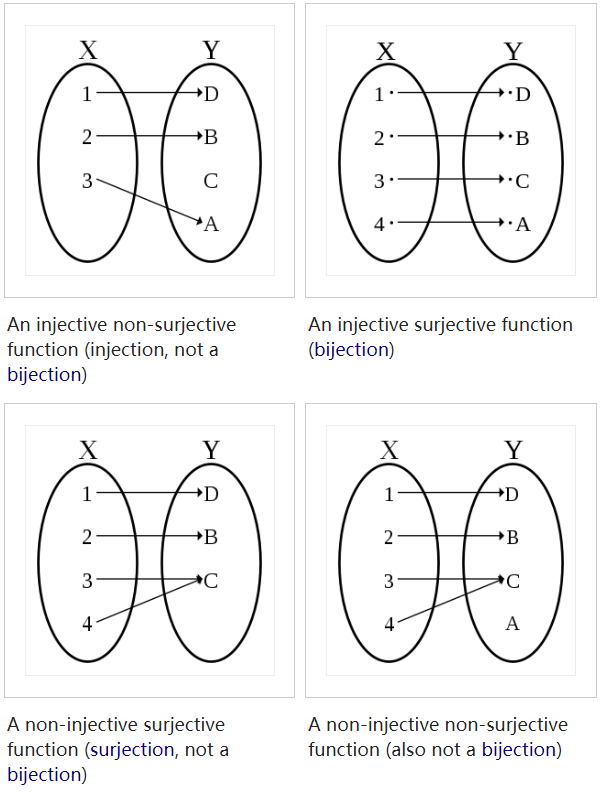### Complex

• $\lvert z \rvert = \sqrt{x^2 + y^2} = \rho$ the length of the complex number z
• $x = \rho \cos \theta , \; y = \rho \sin \theta$
$z = \rho (\cos \theta + i \sin \theta)$
• Crucial identity
$e^{i\theta}= \cos \theta + i \sin \theta$
$z = \rho e^{i\theta} ,\; \rho > 0 ,\; \theta$ real
$e^{i\theta + 2i\pi m} = e^{i\theta},\; m \in \mathbb{Z}$

### Function Space

• $C^0 (U) = \lbrace u: U \to \mathbb{R} \mid u$ continuous }
• $C (\bar{U}) = \lbrace u: \in C(U) \mid u$ uniformly continuous }
• $C^k (U) = \lbrace u: U \to \mathbb{R} \mid u$ is k-times continuously differentiable }
• $C^\infty (U) = \cap_{k=0}^{\infty} C^k (U) = C^\infty (\bar{U}) = \cap_{k=0}^{\infty} C^\infty (\bar{U})$
• $L_p \mathrm{or} L^p$ function space
• $L_p (U) = \lbrace u : U \to \mathbb{R} \mid \lvert u \rvert_{L^p(U)} < \infty \rbrace$ where u is Lebesgue measurable
• $\lVert u \rVert_{L^p(U)} = \displaystyle (\int_{U} \lvert f \rvert^p dx )^{\frac{1}{p}}, (1< p < \infty)$

### Convolution

• If $f, g : \mathbb{R}^n \to \mathbb{C}$, then the convolution is :
• $(f \star g)(x) = \displaystyle \int_{\mathbb{R}^n} f(y) g(x-y) dy$
• If $f, g, h \in C_0^\infty (\mathbb{R}^n)$
• $f \star g = g \star f$
• $f \star (g \star h) = (f \star g) \star h$
• $\displaystyle \int_{\mathbb{R}^n} (f \star g)(x) dx = \displaystyle \int_{\mathbb{R}^n} f (x) dx \displaystyle \int_{\mathbb{R}^n} g (x) dx$
• Support $f \in L_{loc.}^1 (\mathbb{R}^n), g \in C_0^k (\mathbb{R}^n)$ for some $k \geq 0$, then $(f \star g) \in C^k(\mathbb{R^n})$ and
• $D^\alpha(f \star g) = f \star D^\alpha g , \lvert \alpha \rvert \leq k$

### Null Sequence

• Null sequence $\lbrace \sigma_j \rbrace_{j \in \mathbb{N}}$ is a sequence converges to a limit of 0:
• $\displaystyle \lim_{j \to \infty} \sigma_j = 0$

## Theorems and Definitions

### Morphism

• A category $\mathsf{C}$ consists of
• a class $Obj(\mathsf{C})$ of objects of the category
• for every two objects $A$, $B$ of $\mathsf{C}$, a set $Hom_\mathsf{C} (A, B)$ of morphisms, with the properties below:
1. For every object $A$ of $\mathsf{C}$,
$\exists I_A \in Hom_\mathsf{C} (A, A)$ the identity of $A$
2. One can compose morphism:
Two morphisms $f \in Hom_\mathsf{C}(A, B)$ and $g \in Hom_\mathsf{C}(B, C)$ determine a morphism $gf \in Hom_\mathsf{C}(A, C)$
There is a function (of sets)
$Hom_\mathsf{C}(A, B) \times Hom_\mathsf{C}(B, C) \to Hom_\mathsf{C}(A, C)$
and the image of the pair $(f, g)$ is denoted $g f$
3. Associative: $h \in Hom_\mathsf{C}(C, D)$
$(hg)f = h(gf)$
4. Identity morphisms are identities
$f I_A = f, \; \; I_B f = f$
5. the sets $Hom_\mathsf{C} (A, B), \; Hom_\mathsf{C} (C, D)$ be disjoint unless $A = C, B = D$
• Homomorphism
A homomorphism is a map between two algebraic structures of the same type, that preserves the operations of the structures
Formally, a map $f:A\to B}$ preserves an operation $\mu$ of arity $k$, defined on both $A$ and $B$ if
$\displaystyle f(\mu_{A}(a_{1},\ldots ,a_{k}))=\mu_{B}(f(a_{1}),\ldots ,f(a_{k})),$
for all elements $a_1, …, a_k \in A$

• Endomorphism
A morphism od an object $A$ of a category $\mathsf{C}$ to itself
$Hom_\mathsf{C}(A, A)$ is denoted $End_\mathsf{C} (A)$

• Mono-morphism (or Monic)
• A function $f : A \to B$ is a mono-morphism (or monic) if holds:
for all sets $Z$ and all functions $\alpha^\prime, \alpha^{\prime\prime} : Z \to A$
(for all objects $Z$ of $\mathsf{C}$ and all morphisms $\alpha^\prime, \alpha^{\prime\prime} \in Hom_\mathsf{C}(Z, A)$ )
$f \circ \alpha^\prime = f \circ \alpha^{\prime\prime} \to \alpha^\prime = \alpha^{\prime\prime}$
• A function is injective iff it is a monomorphism
• Epimorphism
• for all objects $Z$ of $\mathsf{C}$ and all morphisms $\beta^\prime, \beta^{\prime\prime} \in Hom_\mathsf{C}(B, Z)$
$\beta^\prime \circ f = \beta^{\prime\prime} \circ f \to \beta^\prime = \beta^{\prime\prime}$
• A function is surjective iff it is epimorphism
• Isomorphism
• A morphism $f \in Hom_\mathsf{C} (A, B)$ is isomorphism if it has a (two sided) inverse under composition: that is
if $\exists g \in Hom_\mathsf{C}(B, A)$ s.t. $gf = I_A, fg = I_B$
• The inverse of an isomorphism is unique
• prop.
• Each identity $I_A$ is an isomorphism and is its own inverse
• If $f$ is an isomorphism, then $f^{-1}$ is also and $(f^{-1})^{-1} = f$
If $f, g$ are isomorphisms, the $gf$ is also and $(gf)^{-1} = f^{-1}g^{-1}$
• Endomorphism
An endomorphism is a homomorphism whose domain = codomain, or
A morphism whose source is equal to the target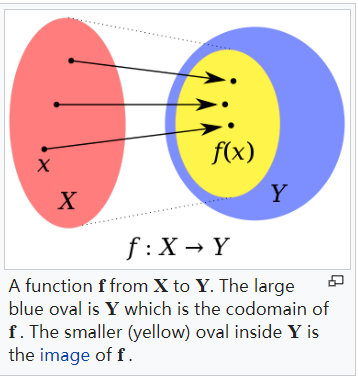• Automorphism
An automorphism is an endomorphism also an isomorphism

### Normed Vector Space

• Normed Vector Space is a vector space (v.s.) V with a norm $\lVert \cdot \rVert \ : \ V \to \mathbb{R}, \ v \mapsto \lVert v \rVert$ satisfying:
1. $\lVert v \rVert \geqslant \ \forall \ v \in V \ \mathrm{and} \ \lVert v \rVert = 0 \ \mathrm{iff} \ v = 0$ (pos. def)
2. $\lVert \lambda v \rVert = \lvert \lambda \rvert \lVert v \rVert$ for every $\lambda \in \mathbb{k}$ and $v \in V$ (pos. homogeneity)
3. $\lVert v \ + \ w \rVert \ \leqslant \ \lVert v \rVert + \lVert w \rVert$ for every $v, w \in V$ (triangle ineq.)
• If $V$ is a separable normed space, then $V$ embeds isometrically into $l_\infty$

### Vector Space Operation

• Vector Space Operations are continuous maps
• $\mathbb{K} \times V \to V , (\lambda , v) \mapsto \lambda v$ (scalar multi.)
• $V \times V \to V , (v , w) \mapsto v + w$ (addition)

### Topological Vector Space

• Topological Vector Space (top.) is a vector space together with a topology which makes the vector space operations continuous and points are closed

### Bounded

• Let V be a topological vector space and $B \subset V$
We say that B is bounded if
for every open neighbourhood u of 0, there exists $t > 0$
s.t. $sU \supset B \; \forall \; s \geq t$

### Banach Space

• a Banach Space is a normed vector space that is complete as a metric space
i.e. every Cauchy sequence converges
• Let V be a normed space and W a Banach space. Then $\mathfrak{B} (V, W)$ is a Banach space
• Let V be a normed v.s. then $V^{\star}$ is a Banach space
• Vector-valued Liouville’s Theorem
Let X be a comple Banach Space, and $f: \mathbb{C} \to X$ a bounded analytic function
then $f$ is constant

### Linear Map

• In any topological vector spaces V, W ;
a linear map $T: V \to W$ is countinuous iff it is countinuous at 0

• T is bounded if $T(B)$ is bounded for any bounded $B \subset V$

Fact.

• If V,W are normed v.s., a linear map $T : V \to W$ is bounded iff
there is a $\lambda > 0$ s.t. $T (B_1(0)) \subseteq B_\lambda (0)$
i.e. $\lVert TV \rVert \leq \lambda \; \forall \; v \in V$ with $\lVert v \rVert \leq 1$

### Operator Norm

• Let V,W be normed v.s.
The operator norm of a linear map $T : V \to W$ is
$% $
• Denote by $\mathfrak{L} (V, W)$ the space of linear maps $V \to W$
by $\mathfrak{B} (V, W)$ the space of bounded linear maps $V \to W$

Fact.

• The operator norm $\lVert \cdot \rVert$ is a norm on $\mathfrak{B} (V, W)$

Prop.

• Let V,W be normed v.s.
Then a linear map $T : V \to W$ is bounded iff it is countinous

### Dual Space

• Let V be a top. v.s.
The $V^{\star}$ (top.) dual space of V is the space of all continuous linear maps $V \to \mathbb{K}$
• We call $\mathfrak{L} (V, \mathbb{k})$ the algebraic dual of V
• Let V be a normed v.s.
The double dual of V is the dual space of $V^{\star}$
i.e. $V^{\star \star} = (V^{\star})^{\star}$
$V^{\star\star}$ is also called bidual or the second dual of $V$
For each $x \in X$, define $\hat{x} : X^\star \to$ scalars by $\hat{x}(f) = f(x)$
then $\hat{x}$ is linear, and $\lvert \hat{x} (f) \rvert = \lvert f(x) \rvert \leq \lVert f \rVert \cdot \lVert x \rVert$
so $\hat{x} \in X^{\star\star}$ with $\lVert \hat{x} \rVert \leq \Vert f \rVert$

• If X be a normed space
The dual space $X^\star$ is the space of all bounded linear functionals on $X$
This is a normed space with norm $\lVert f \rVert = \sup{\lvert f(x) \rvert : x \in B_X }$ , where $B_X = { x \in X : \lVert x \rVert \leq 1}$
$X^\star$ is always complete

Fact.

• The map $\phi : V \to V^{\star \star}$ , $v \mapsto \tilde{v}$ where $\tilde{v} (f) = f(v)$ is bounded and linear
• A Banach space is reflexive if $\phi$ is bijection

• Dual operators

Define the dual operator of $T$ by $T^\star : X^\star \to Y^\star , T^\star (g) = g \circ T$ for $g \in Y^\star$
This is well-defined as $g \circ T$ is a composition of bounded linear operators
Furthermore, $T^\star$ is linear and bounded, since
%

### Topologies on Banach Spaces

• Let $(X, \lvert . \rvert)$ denote a real Banach space
• $\displaystyle X^\star = { l : X \to R \ \mathrm{linear \ s.t. } \lvert l \rvert_{X^\star} = \sup_{x\neq 0} \frac{\lvert l(x) \rvert}{\lvert x \rvert_X} \leq \infty }$
• Topologies on $X$
1. The strong topology $x_n \displaystyle \longrightarrow_{X} x$ defined by
$\lvert x_n - x \rvert_X \to 0 \ (n \to +\infty)$
2. The weak topology $x_n \displaystyle \longrightarrow_{X} x$ defined by
$l(x_n) \to l(x) \ (n \to +\infty)$ for every $l \in X^\star$
• Topologies on $X^\star$
1. The strong topology $l_n \displaystyle \longrightarrow_{X} l$ defined by
$\lvert l_n - l \rvert_{X^\star} \to 0 \ (n \to +\infty)$
or equivalently,
$\sup_{x\neq 0} \frac{\lvert l_n(x) - l(x) \rvert}{\lvert x \rvert_X} \to 0 \ (n \to +\infty)$
2. The weak topology $x_n \displaystyle \longrightarrow_{X} x$ defined by $l(x_n) \to l(x) \ (n \to +\infty)$ for every $l \in X^\star$

• Let V,W be normed v.s. and $T \in \mathfrak{B}(V, W)$
Then the adjoint map $T^{\star} : W^{\star} \to V^{\star}$ is defined by
$[T^{\star} f] v = f (T v) \; \mathrm{for} \; f \in W^{\star}, v \in V$

Fact.

• $T^\star f$ is indeed in $V^\star = \mathfrak{B} (V, \mathbb{K})$ and $\lVert T^\star \rVert \leq \lVert T \rVert$

### Finite Dimentional

• Any finite-dimensional vector space can be identified with $\mathbb{K}^n$ by choosing a basis. there n is the dimension
• Two norms $\lVert \cdot \rVert$ and $\lvert \lVert \cdot \rVert \rvert$ on a vector space V are equivalent iff
there exists a constant $C > 0$ s.t.
$C^{-1} \lvert \lVert v \rVert \rvert \leq \lVert v \rVert \leq C \lvert \lVert v \rVert \rvert$ for all $v \in V$

Prop.

• All norms on a f.d.v.s. are equivalent
• In any f.d.v.s., the closed unit ball is compact
• Every f.d. normed v.s. is a Banach space
• Let V be a normed v.s. and $W \subset V$ be a f.d. subspace, then W is closed

Thm.

• Let V be a normed v.s. s.t. $\overline{B_1(0)}$ is compact. Then V is finite-dimentional

### Sublinear

• a map $p : V \to \mathbb{R}$ is sublinear if
1. $p( \alpha v ) \ = \ \alpha \ p(v) \; \forall v \in V , \ \alpha \geq 0$
2. $p(v+w) \ \leq \ p(v) + p(v) \; \forall v,\ w \in V$

### Poset

• A partially ordered set (poset) is a set P with a binary relation $\leq$ s.t. for all $x, y \in P$ either $x \leq y$ or $x \nleq y$ , and
$x \leq x$ (reflexive)
$x \leq y , y \leq z \; \Rightarrow \; x \leq z$ (transitive)
$x \leq y , y \leq x \; \Rightarrow \; x = y$ (antisymmetric)

• Let P be a poset. An element $m \in P$ is maximal if $m \leq x \to m = x$

### Totally Ordered

• Let P be a poset. a subset $T \subset P$ is called totally ordered (or a chain) if
$x \nleq y \Rightarrow y \leq x$
i.e.
either $x \leq y or y \leq x$

### Linearly Independent

• In a v.s. V, elements $v_1, \cdots , v_k$ are linearly independent if
$\sum_{i = 1}^{k} \alpha_i v_i = 0 \; \to \; \alpha_1 = \alpha_2 = \cdots = 0$
• a set $S \subset V$ is linearly independent if any finite subset is
• A basis of V is a set $B \subset V$ that is linearly independent and such that every element of V is a finite linear combination of elements in B

Prop.

• Let $V \neq \lbrace 0 \rbrace$ be a vector space and $S \subset V$ linearly independent. Then V has a basis B s.t. $S \subset B$

### Extend

• Given vector spaces $W \subset V$ , linear maps $g : W \to \mathbb{K} , f : V \to \mathbb{K}$ , we say that f extends g if $f \lvert_{w} = g$

### Hahn Banach

• Let V be a real vector space, a subspace $W \subset V , p : V \to \mathbb{R} \; \mathrm{sublinear}, g: W \to \mathbb{R}$ linear s.t.
$g(v) \leq p(v) \; \forall v \in W$
Then there is $f : V \to \mathbb{R}$ linear s.t. $f\lvert_W = g$ and
$f(v) \leq p(v) \; \forall v \in V$

Cor.

• Let $V \in \mathbb{K}$ be a normed v.s., $W \subset V$ a subspace
For any $g \in W^\star$ there exists $f\in V^{star}$
s.t. $f\lvert_{W} = g$ and $\lVert f \rVert \leq \lVert g \rVert$
• Let V be a normed vector space and $v \in V$
Then $\exists f_v \in V^\star$ s.t. $\lVert f_v \rVert = 1$ and $f_v (V) = \lVert v \rVert$
• Here $f_v$ is called a support functional for V
• Let V be a normed v.s. and $v \in V$ , then
$v = 0 \Leftrightarrow f(v) = 0 \; \forall \; f \in V^\star$
In particular, $V^\star \neq 0$
• Let V be a normed v.s., $v, w \in V , \ v \neq w$ . Then
there exists $f \in V^\star$ s.t. $f(v) \neq f(w)$

Prop.

• The map $\phi : V \to V^{\star \star }$ is an isometry
($d_Y(f(x),f(y)) = d_X(x,y)$ for a map $f: X \to Y$) that
$\lVert \phi (v) \rVert = \lVert v \rVert$
• Let V, W be a normed v.s.
For any $T \in \mathfrak{B} (V, W)$ , the dual map $T^\star \in \mathfrak{B} (W^\star, V^\star )$ satisifies $\lVert T^\star \rVert = \lVert T \rVert$

### Support

• If the domain of f is a top. space, the support of f is the smallest closed set containing all points not mapped to zero

Closed Support:

• The support of f is defined topologically as the closure of the subset of X where f is non-zero
• support of a function $f : X \to \mathbb{C}$ is the closure of the set $\lbrace x \in X \mid f(x) \neq 0 \rbrace$; it is the closed subset of X
i.e. $supp(f) := \overline{\lbrace x \in X \mid f(x) \neq 0 \rbrace}$ $= \overline{f^{-1}(\lbrace 0 \rbrace^c)}$

Compact Support:

• The closed support is a compact subset of $X$
• If $X$ is the real line, or n-dimensional Euclidean space, then a function has compact support iff it has bounded support
• Each function is nonzero on a closed and bounded interval, but is zero everywhere else

Essential Support:

• If X is a top. measure space with a Borel measure μ
the essential support of a measurable function $f : X \to \mathbb{R}$, written ess supp(f), is defined to be the smallest closed subset $F$ of $X$ such that $f = 0$ μ-almost everywhere outside $F$
Equivalently, ess supp(f) is the complement of the largest open set on which f = 0 μ-almost everywhere $\displaystyle \mathrm{ess supp}(f) := X \setminus \bigcup \lbrace \Omega \subset X \mid \Omega$ is open and $f = 0$ μ-almost everywhere in $\Omega \rbrace$

Support Function:

• The support function $h_A : \mathbb{R}^n \to \mathbb{R}$ of a non-empty closed convex set A in $\mathbb{R}^n$ is given by
$h_A (x) = sup \lbrace x \cdot a : a \in A \rbrace , \; x \in \mathbb{R}^n$

Supporting Functional:
In convex analysis and mathematical optimization, the supporting functional is a generalization of the supporting hyperplane of a set

• Let X be a locally convex top. space and $C \subset X$ be a convex set, then
the continuous linear functional $\phi : X \to \mathbb{R}$ is a supporting functional of C at the point $x_0$
if $\phi(x) \leq \phi(x_0)$ for every $x \in C$
• If $h_C : X^\star \to \mathbb{R}$ is a support function of the set C, then
if $h_C (x^\star) = x^\star (x_0)$ , it follows that
$h_C$ defines a supporting functional $\phi : X \to \mathbb{R}$ of $C$ at the point $x_0$ s.t.
$\phi(x) = x^\star (x)$ for any $x \in X$
• If $\phi$ is a supporting functional of the convex set C at the point $x_{0} \in C$ such that
$\displaystyle \phi (x_{0}) = \sigma = \sup_{x \in C} \phi (x) > \inf_{x \in C} \phi (x)$
then $H = \phi^{-1} (\sigma )$ defines a supporting hyperplane to C at $x_{0}$

### Dense

• If X is a metric space, the $Y \subset X$ is dense if $\overline{Y} = X$
i.e. $Y \cap B_r (X) \neq \varnothing \; \forall \; x \in X , r > 0$

### Baire Category Theorem

• Let X be a complete metric space.
For any sequence of open dense subsets $U_j \subset X$ , $\displaystyle\bigcap_j U_j$ is dense in $X$

cor.

• Let $X$ be a complete metric space. Let $A_j \subset X$ be a sequence of closed subsets s.t.
$\bigcup_j A_j$ has nonempty interior, i.e. it contains some ball
Then at least one of the $A_j$ has nonempty interior

### Interior

• The point x is an interior point of S. The point y is on the boundary of S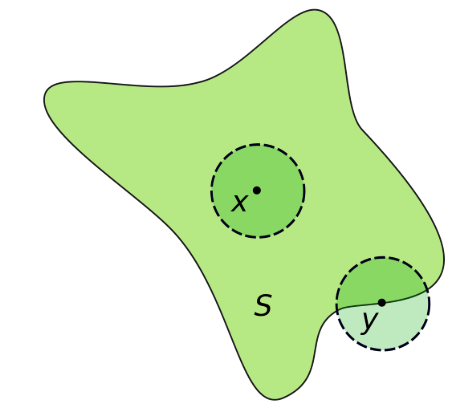• Interior point
If $S$ is a subset of a Euclidean space, then $x$ is an interior point of $S$ if there exists an open ball centered at $x$ which is completely contained in S
• Interior of a set
The interior of a set $S$ is the set of all interior points of $S$
it is denoted $int(S)$, $Int(S)$ or $S^o$
and has the following properties:
• $int(S)$ is an open subset of $S$
• $int(S)$ is the union of all open sets contained in $S$
• $int(S)$ is the largest open set contained in $S$
• A set $S$ is open iff $S = int(S)$
• $int(int(S)) = int(S)$ (idempotence)
• If $S$ is a subset of $T$, then $int(S)$ is a subset of $int(T)$
• If $A$ is an open set, then $A$ is a subset of $S$ iff $A$ is a subset of $int(S)$

### Meagre

• Let X be a metric space
1. A subset $Y \subset X$ is nowhere dense if $int( \overline{Y} ) = \varnothing$ ,
( if the interior of its closure is empty )
i.e. , if $Y$ is not dense in any ball
2. A subset $Z \subset X$ is meagre or of the first category if
there are countably many set $Y_j \subset X$ that are nowhere dense and $Z = \bigcup_j Y_j$
( if it is a union of countably many nowhere dense subsets )
3. A subset $U \subset X$ is nonmeagre or of the second category if it is not meagre
( it contains a countable union of open and dense sets )
4. A subset $R \subset X$ is residual if
its complement is meagre

fact.

• $Y \subset X$ is nowhere dense
$\Leftrightarrow \; \overline{Y}$ is nowhere dense
$\Leftrightarrow \; X \backslash \overline{Y}$ is open dense

Cor.

• Let $X$ be a complete metric space. Then $X$ is of second category
• Let $X$ be a complete metric space. Then residual sets are non-meagre and dense
• Let $X$ be a complete metric space and $U \subset X$ open.
Then $U = \varnothing$ or $U$ is of the second category

### Uniform Boundedness

• Principle of uniform boundedness
Let X be a complete metric space
Let $(f_\lambda)\mid_{\lambda \in \wedge}$ be a family of continuous functions $f_\lambda : X \to \mathbb{R}$
If $(f_\lambda)\mid_{\lambda \in \wedge}$ is pointwise bounded,
i.e., $\sup_{\lambda \in \wedge} \lvert f_\lambda (x) \rvert \leq \infty$ for every $x \in X$,
then there is a ball $B_r (X_c) \subset X$ s.t.
$( f_\lambda )$ is uniformly bounded on $B_r(X_0)$
i.e., $\sup_{\lambda \in \wedge} \sup_{x \in B_r (x_0)} \lvert f_\lambda (x) \rvert \leq \infty$

• Banach-Steinhaus Theorem
Let $V$ be a Banach Space and $W$ a normed v.s.
Let $(T_\lambda)\mid_{\lambda \in \wedge} \subset \mathfrak{B}(V,W)$ be pointwise bounded,
i.e., $\sup_{\lambda \in \wedge} \lVert T_\lambda v \rVert \; \forall \; v \in V$
Then $( T_\lambda)$ is uniformly bounded
i.e., $\sup_{\lambda \in \wedge} \lVert T_\lambda \rVert \leq \infty$

### Open Mapping Theorem

• A map between topological spaces is open iff it maps open ses to open sets

• Open Mapping Theorem
Let $V, W$ be Banach Spaces and $T \in \mathfrak{B} (V, W)$

1. if $T$ is surjective, then $T$ is open
2. if $T$ is bijective, then $T^{-1} \in \mathfrak{B}(W, V)$

### Fubini’s theorem

• If $f(x,y)$ is $X \times Y$ integrable, that $f(x,y)$ is measrable function and $\displaystyle \int_{X \times Y} \lvert f(x,y) \rvert d(x,y) < \infty$ then
• $\displaystyle \int_{X} (\int_{Y} f(x,y)dy)dx = \int_{Y}(\int_{X} f(x,y) dx) dy = \int_{X \times Y}f(x,y) d(x,y)$

# Boundary Value Problems for Linear PDEs

## Complex Variables

### Analytic

• $f(z) = u(x, y) + iv(x, y)$ where $u , v$ are real functions
We called $f(z)$ an analytic at point $z_0$
if it is differentiable in a neighbourhood of $z_0$
• $f(z)$ is analytic iff functions $u , v$ satisfy Cauchy–Riemann equations
• $f$ is an analytic function iff dbar-Derivative
$\displaystyle \frac{\partial f}{\partial \bar{z}} = 0$ or $\displaystyle \frac{\partial f}{\partial z} = u_x - i u_y = v_y + i v_x$

• Liouville’s theorem:
If $f(z)$ is everywhere analytic (in the finite complex plane) and bounded (including infinity), then $f(z)$ is a constant.

• Rouche’s theorem:
Let $f(z)$ and $g(z)$ be analytic on and inside a simple contour $C$.
If $|f(z)| > |g(z)|$ on $C$, then $f(z)$ and $f(z) + g(z)$ have the same number of zeros inside the contour $C$.

• Morera’s theorem:
If $f(z)$ is continuous in a domain $D$ and if
$\displaystyle \oint_C f(z) dz = 0$
for every simple closed contour $C$ lying in $D$,
then $f(z)$ is analytic in $D$.

• Maximum Principle:
If $f(z)$ is analytic in a bounded region $D$
and $\lvert f(z) \rvert$ is continuous in the closed region $\bar{D}$,
then $\lvert f(z)\rvert$ obtains its maximum on the boundary of the region

• Minimum Principle:
If, in addition, it is non-zero at all points of $D$, then $\lvert f(z) \rvert$ obtains its minimum on the boundary of the region.

• Laurent Series:
A function $f(z)$ analytic in an annulus(环) $R_1 < \lvert z - z_0 \rvert < R_2$ may be presented by the expansion
$f(z) = \displaystyle\sum_{m=-\infty}^{\infty} A_m(z-z_0)^m$
where $A_m = \displaystyle \frac{1}{2i\pi} \oint_C \frac{f(z)dz}{(z-z_0)^{m+1}}$
and $C$ is any simple closed contour in the region of analyticity enclosing the inner boundary $\lvert z - z_0 \rvert = R_1$.

### Cauchy Riemann equations

• The Cauchy–Riemann equations on a pair of real-valued functions of two real variables $u(x,y)$ and $v(x,y)$ are the two equations:
1. $\displaystyle \frac{\partial u}{\partial x} = \frac{\partial v}{\partial y}$
2. $\displaystyle \frac{\partial u}{\partial y} = - \frac{\partial v}{\partial x}$
That $u_x = v_y , \; u_y = - v_x$

### Holomorphic

• Holomorphy is the property of a complex function of being differentiable at every point of an open and connected subset of $C$ (a domain in $C$)
• If f is complex-differentiable at every point $z_0$ in an open set $U$, we say that $f$ is holomorphic on $U$.
We say that $f$ is holomorphic at the point $z_0$ if it is holomorphic on some neighbourhood of $z_0$.
• In complex analysis, a function that is complex-differentiable in a whole domain (holomorphic) is the same as an analytic function
NOT true for real differentiable functions
• Any holomorphic function is actually infinitely differentiable and equal to its own Taylor series (analytic)

### dbar Derivative

• the dbar-derivative with respect to $\bar{z}$
i.e. the derivative with respect to the complex conjugate of $z$
The equations $z = x + iy , \; \bar{z} = x - iy$
imply $x = \displaystyle \frac{z+\bar{z}}{2} , \; y = \frac{z-\bar{z}}{2i}$
Chain rule yields
$\displaystyle \frac{\partial}{\partial z} = \frac{1}{2} (\frac{\partial}{\partial x}-i\frac{\partial}{\partial y}) , \; \displaystyle \frac{\partial}{\partial \bar{z}} = \frac{1}{2} (\frac{\partial}{\partial x}+i\frac{\partial}{\partial y})$

### Cauchy’s Theorem

Cauchy integral theorem (also known as the Cauchy–Goursat theorem)

• Suppose that $f(z)$ is analytic in the domain $D$.
Then, the integral of $f(z)$ along the boundary of $D$ vanishes.

### Green’s Theorem

• Let $C$ be a positively oriented, piecewise smooth, simple closed curve in a plane, and let $D$ be the region bounded by $C$.
If $L$ and $M$ are functions of $(x, y)$ defined on an open region containing $D$ and have continuous partial derivatives there, then
$\displaystyle \oint_C (L dx + M dy) = \int \int_D (\frac{\partial M}{\partial x} - \frac{\partial L}{\partial y}) dx dy$
where the path of integration along C is anticlockwise

### Residue Theorem

• Suppose that the function $f(z)$ is analytic in the domain $D$,
except at the single point $z_0$ where the function has a pole with residue $g(z_0)$, namely in the neighborhood of $z_0$, this function can be written as
$f(z) = \displaystyle \frac{g(z)}{z - z_0}$ where $g(z)$ is analytic.
Then, the integral of $f(z)$ along the boundary of $D$ equals $2i\pi g(z_0)$

• Cauchy’s Residue Theorem

• $\oint_C f(z)dz = 2 \pi i \displaystyle \sum_{k = 1}^n \mathrm{Res} (f(z), z_k)$
• If $z_k$ is a pole of order k, then the residue of f around $z = z_k$ can be found by the formula:
Res $(f , z_k) = \displaystyle \frac{1}{(k-1)!} \lim_{z\to z_k} \frac{d^{k-1}}{dz^{k-1}} ((z-z_k)^k f(z))$
• For essential singularity case, the residues must usually be taken directly from series expansions

### Taylor Series

• Analytic real function can be expanded in terms of an infinite series:
$f(x) = \displaystyle \sum_{m=0}^\infty \frac{(x-x_0)^m}{m!} \frac{d^m}{dx^m} f(x_0)$
where this series is valid provided that $\lvert x - x_0 \rvert < R$ and $R$ is called the radius of convergence

• Complexification
Let $f(z)$ be analytic for $\lvert z - z_0 \rvert < R$
Then $f(z) = \displaystyle \sum_{m=0}^\infty \frac{(z-z_0)^m}{m!} \frac{d^m}{dz^m} f(z_0)$
implies $f(\zeta) = \displaystyle \frac{1}{2i\pi}\oint_{\partial D} \frac{f(z)dz}{z-\zeta}$
implies $\displaystyle \frac{d^m}{d\zeta^m} f(\zeta) = \frac{m!}{2i\pi}\oint_{\partial D} \frac{f(z)dz}{(z-\zeta)^{m+1}}$

### Principal Value integrals:

• Let $0 < a < b$ and $z_0 \in (a, b)$ be a pole of $f(z)$.
The principal value integral is given as
$\displaystyle PV\int_a^b f(z) dz = \lim_{\epsilon \to 0} (\int_{a}^{z_0 - \epsilon} + \int_{z_0 + \epsilon}^{b}) f(z) dz$
This notion is extended to integration over curves in the complex plane.
• In the simplest cases equip the above contour of integration with a small semicircle center at $z_0$ and radius $\epsilon$, denoted by $C_\epsilon$ . Then using the parametrisation $z_0 + \epsilon e^{i\theta}$ and letting $\epsilon \to 0$ , compute the contribution of this pole

### Jordan Lemma

• Let $C_R$ denote the semi-circle of radius $R$ in the upper half complex $z$-plane centered at the origin.
Assume that the analytic function $f(z)$ vanishes on $C_R$ as $R \to \infty$ , namely
$\lvert f(z) \rvert < K (R),\; z \in C_R$
and $K(R) \to 0$ as $R \to \infty$
Then, $\displaystyle\int_{C_R} e^{iaz} f(z) dz \to 0$ as $R \to \infty$ for $a > 0$

ExerciseB01 $\int_{-\infty}^{+\infty} = \displaystyle \frac{sinx}{x}$

### Fourier Transform

• Fourier Transforms
• The Fourier Transform of a function $f(x) , \; -\infty < x < \infty$ , is defined by
$\hat{f}(\lambda) = \displaystyle \int_{-\infty}^{\infty} e^{-i\lambda x} f(x) dx, \; -\infty < \lambda < \infty$

• The Inverse Fourier Transform of a function $f(\lambda) , \; -\infty < \lambda < \infty$ , is defined by
$\tilde{f}(x) = \displaystyle \frac{1}{2\pi} \int_{-\infty}^{\infty} e^{i\lambda x} \hat{f}(\lambda) d\lambda, \; -\infty < x < \infty$

• In order to be well-defined, we need that $f(x) \in L_2$ that is
$\displaystyle \int_{-\infty}^{\infty} \lvert f(x) \rvert ^2 dx < \infty$

• Fourier transform of derivative
$\widehat{f^{(n)}} (\lambda) = (i\lambda)^n \hat{f}(\lambda)$

# Inverse Problems in Imaging

This note is taken from the lecture notes in the Part III course of University of Cambridge in 2019
Source of the notes:
Wikipedia
Inverse Problems in Imaging notes

## Notation

• Let $A$ be bounded linear operators

• $A \in \mathcal{L}(\mathcal{U}, \mathcal{V})$ with
$\displaystyle \lVert A \rVert_{\mathcal{L}(\mathcal{U}, \mathcal{V})} := \sup_{u \in \mathcal{U} \backslash \lbrace 0 \rbrace} \frac{\lVert A u \rVert_\mathcal{V}}{\lVert u \rVert_\mathcal{U}} = \sup_{\lVert u \rVert_\mathcal{U} \leq 1} \lVert Au \rVert_\mathcal{V} \leq \infty$
• For $A : \mathcal{U} \to \mathcal{V}$
• $\mathsf{D}(A) := U$ the domain
• $\mathsf{N}(A) := \lbrace u \in \mathcal{U} \mid Au = 0 \rbrace$ the kernel
• $\mathsf{R}(A) := \lbrace f \in \mathcal{V} \mid f = Au, u \in \mathcal{U} \rbrace$ the range
• A is continuous at $u \in \mathcal{U}$
• if $\forall ε > 0 \exists δ > 0$ with
$\lVert Au - Av \rVert_\mathcal{V} \leq ε \ \forall \ v\in \mathcal{U} \ \mathrm{with}\ \lVert u - v \rVert_\mathcal{U} \leq δ$
• $A^*$ the (unique) adjoint operator of $A$ with
• $\langle Au, v \rangle_\mathcal{V} = \langle u, A^*v \rangle_\mathcal{U} \ \forall \ u \in \mathcal{U}, v \in \mathcal{V}$
• For a subset $\mathcal{X} \subset \mathcal{U}$, the orthogonal complement of $\mathcal{X}$ is defined by
• $\mathcal{X}^\perp := \lbrace u \in \mathcal{U} \mid \langle u,v\rangle_\mathcal{U} = 0 \ \forall \ v \in \mathcal{X} \rbrace$
• Let $A \in \mathcal{L}(\mathcal{U},\mathcal{V})$. Then $A$ is saide to be compact if for any bounded set $B \subset \mathcal{U}$ the closure of its image $\overline{A(B)}$ is compact in $\mathcal{V}$. We denote the spave of compact operators by $\mathcal{K}(\mathcal{U},\mathcal{V})$.

## Introduction

• Operator equations
$Au = f$
where $A : \mathcal{U} \to \mathcal{V}$ is the forward operator acting between some spaces
$\mathcal{U}$ and $\mathcal{V}$ , typically Hilbert or Banach Spaces
$f$ are the measured data
$u$ is the quantity we want to reconstruct from data

### Well-Posed

• The well-posed problem if
1. it has a solution $\forall f \in \mathcal{V}$
2. the solution is unique
3. the solution depends continuously on the data i.e. small errors in the data f result in small errors in the reconstruction

### Differentiation

• Evaluation the derivative of a function $f \in L^2 [0, \pi /2 ]$
$D f = f^\prime$
where $D : L^2 /[ 0, \pi /2 /] \to L^2 [ 0, \pi /2 ]$

• Prop The operator D is unbounded from $L^2 /[ 0, \pi /2 /] \to L^2 [ 0, \pi /2 ]$

• Integral transform of the image:
$f(x) = (Au) (x) := \int K(x,\xi) u(\xi) d\xi ,$
$u(\xi)$ is the “true” image
$K(x,\xi)$ is the point-spread function (PSF) which models the optics of the camera

Fatou’s lemma

## Generalised Solutions

### Orthogonal decomposition

• For $A \in \mathcal{L}(\mathcal{U},\mathcal{V})$, we have
• $\mathsf{R}^\perp(A) = \mathsf{N}(A^* )$ then $\overline{\mathsf{R}(A)} = \mathsf{N}(A^* )^\perp$
• $\mathsf{R}^\perp(A^* ) = \mathsf{N}(A)$ then $\overline{\mathsf{R}(A^* )} = \mathsf{N}(A)^\perp$
• Hence, we can deduce the following orthogonal decompositions
• $\mathcal{U} = \mathsf{N}(A) \oplus \overline{\mathsf{R}(A^* )}$
• $\mathcal{V} = \mathsf{N}(A^* ) \oplus \overline{\mathsf{R}(A)}$
• Lemma 1. Let $A \in \mathcal{L}(\mathcal{U},\mathcal{V})$ . Then $\overline{\mathsf{R}(A^* A)} = \overline{\mathsf{R}(A^* )}$

### Minimal-norm Solutions

• An element $u \in \mathcal{U}$ is called
• a least-squares solution of $Au =f$ if
$\lVert Au - f \rVert_\mathcal{V} = inf\lbrace \lVert Av - f \rVert_\mathcal{V}, v \in \mathcal{U} \rbrace$
• a minimal-norm solution of $Au =f$ (and is denoted by $u^\dagger$ ) if
$\lVert u^\dagger \rVert_\mathcal{U} \leq \lVert v \rVert_\mathcal{U}$ for all least squares solutions $v$
• Thm 1. Let $f \in \mathcal{V} \ \mathrm{and} \ A \in \mathcal{L}(\mathcal{U},\mathcal{V})$. Then the following three assertions are equivalent
1. $u \in \mathcal{U}$ satisfies $Au = P_{\overline{\mathsf{R}(A)}} f$
2. $u$ is a least squares solution of the inverse problem
3. $u$ solves the normal equation
$A^* Au = A^* f$
• Thm 2. Let $f \in \mathsf{R}(A) \oplus \mathsf{R}(A)^\perp$. Then there exists a unique minimal norm solution $u^\dagger$ to the inverse problem and all least squares solutions are given by $\lbrace u^\dagger \rbrace + \mathsf{N}(A)$

### Decomposition of compact operators

• Thm 1. Let $\mathcal{U}$ be a Hilbert space and $A \in \mathcal{K}(\mathcal{U},\mathcal{U})$ be self-adjoint. Then there exists an orthonormal basis $\lbrace x_j \rbrace_{j\in\mathbb{N}} \subset \mathcal{U} \ \mathrm{of} \ \mathsf{R}(A)$ and a sequence of eigenvalues $\lbrace \lambda_j \rbrace_{j\in\mathbb{N}} \subset \mathbb{R}$ with $\lvert \lambda_1 \rvert \geq \lvert \lambda_2 \rvert \geq \cdots > 0$ such that for all $u \in \mathcal{U}$ we have
$Au = \displaystyle \sum_{j=1}^{\infty} \lambda_j \langle u,x_j \rangle_\mathcal{U} x_j$
The sequence $\lbrace \lambda_j \rbrace_{j\in\mathbb{N}}$ is either finite or we have $\lambda_j \to 0$

### Singular Value Decomposition

• the sequence $\lbrace (\sigma_j, x_j, y_j) \rbrace$ is called singular system or singular value decomposition (SVD) of $A$
• Let $A \in \mathcal{K}(\mathcal{U},\mathcal{V})$. Then there exists
1. a not-necessarily infinite null sequence $\lbrace \sigma_j \rbrace_{j\in\mathbb{N}}$ with $\sigma_1 \geq \sigma_2 \geq \cdots > 0$,
2. an orthonormal basis $\lbrace x_j \rbrace_{j\in\mathbb{N}} \subset \mathcal{U} \ \mathrm{of} \ \mathsf{N}(A)^\perp$,
3. an orthonormal basis $\lbrace y_j \rbrace_{j\in\mathbb{N}} \subset \mathcal{V} \ \mathrm{of} \ \overline{\mathsf{R}(A)}$ with
$Ax_j = \sigma_j y_j, \ A^* y_j = \sigma_j x_j, \ \forall \ j \in \mathbb{N}$
• For all $u \in \mathcal{U}$ we have the representation
$Au = \displaystyle \sum_{j=1}^{\infty} \sigma_j \langle u,x_j \rangle y_j$
• For the adjoint operator $A^*$ we have the representation
$A^* f = \displaystyle \sum_{j=1}^{\infty} \sigma_j \langle f,y_j \rangle x_j \ \ \forall \ f\in \mathcal{V}$

### Moore-Penrose inverse

• Let $A \in \mathcal{L}(\mathcal{U},\mathcal{V})$ and let $\widetilde{A} := A\mid_{\mathsf{N}(A)^\perp} : \mathsf{N}(A)^\perp \to \mathsf{R}(A)$ denote the restriction of $A$ to $\mathsf{N}(A)^\perp$
The Moore-Penrose inverse $A^\dagger$ is defined as the unique linear extension of $\widetilde{A}^{-1}$ to
$\mathsf{D}(A^\dagger) = \mathsf{R}(A) \oplus \mathsf{R}(A)^\perp$
with
$\mathsf{N}(A^\dagger) = \mathsf{R}(A)^\perp$.

• Thm 1. Let $A \in \mathcal{L}(\mathcal{U},\mathcal{V})$. Then $A ^\dagger$ is continuous, i.e. $A ^\dagger \in \mathcal{L}(\mathsf{D},\mathcal{U})$, if and only iff $\mathsf{R}(A)$ is closed.

• Lemma 1. The MP inverse $A^\dagger$ satisfies $\mathsf{R}(A^\dagger) = \mathsf{N}(A)^\perp$ and the MP equations
1. $A A^\dagger A = A$ ,
2. $A^\dagger A A^\dagger = A^\dagger$,
3. $A^\dagger A = I - P_{\mathsf{N}(A)}$,
4. $A A^\dagger = P_{\overline{\mathsf{R}(A)}}\mid_{\mathsf{D}(A^\dagger)}$,
where $P_\mathsf{N}$ and $P_\overline{\mathsf{R}}$ denote the orthogonal projections on $\mathsf{N} \ \mathrm{and} \ \overline{\mathsf{R}}$, respectively
• Thm 2. For each $f \in \mathsf{D}(A^\dagger)$, the minimal norm solution $u^\dagger$ to the inverse problem is given via
$u^\dagger = A^\dagger f$.

• Thm 3. Let $A \in \mathcal{K}(\mathcal{U},\mathcal{V})$ with an infinite dimensional range. Then, the MPi of $A$ is discontinuous

• Thm 4. Let $A \in \mathcal{K}(\mathcal{U},\mathcal{V})$ with singular system $\lbrace (\sigma_j, x_j, y_j) \rbrace_{j\in\mathbb{N}}$ and $f \in \mathsf{D} (A^\dagger)$
then the Moore-Penrose inverse of $A$ can be written as
$A^\dagger f = \displaystyle \sum_{j = 1}^{\infty} \sigma_j^{-1} \langle f,y_j \rangle x_j$

• The representation makes it clear that the inverse is unbounded. Indeed, taing the sequence $y_j$ we note that $\lVert A^\dagger y_j \rVert = \sigma_j^{-1} \to \infty$, although $\lVert y_j \rVert = 1$

### Picard criterion

• We say that the data $f$ satisfy the Picard criterion, if
$% $

• Thm 1. Let $A \in \mathcal{K}(\mathcal{U},\mathcal{V})$ with singular system $\lbrace (\sigma_j, x_j, y_j) \rbrace_{j \in \mathbb{N}}$, and $f \in \overline{\mathsf{R}(A)}$. Then $f \in \mathsf{R}(A)$ if and only if the Picard criterion is met

### ill-posed

• An ill-posed inverse problem is mildly ill-posed if the singular values decay at most with polynomial speed, i.e. there exist $\gamma, C > 0$ such that $\sigma_j \geq C j^{-\gamma}$ for all $j$. We call the ill-posed inverse problem severely ill-posed if its singular values decay faster than with polynomial speed, i.e. for all $\gamma, C > 0$ one has that $\sigma_j \leq C j^{-\gamma}$ for $j$ sufficiently large.

## Regularisation Theory

Since the Moore-Penrose inverse of $A$ is unbounded

## Variational Regularisation

The variation formulation of Tikhonov regularisation for some data $f_\delta \in \mathcal{V}$
$\displaystyle\min_{u\in\mathcal{U}} \lVert Au - f_\delta \rVert^2 + \alpha\lVert u \rVert^2$
* fidelity function $\mathcal{F}(Au,f) = \lVert Au - f_\delta \rVert^2$
* regulariser $\mathcal{J}(U) = \lVert u \rVert^2$

the variational regularisation problem is
$\displaystyle\min_{u\in\mathcal{U}} \mathcal{F}(Au,f) + \alpha\mathcal{J}(U)$

### Characteristic function

• Let $\mathcal{C} \subset \mathcal{U}$ be a set.
The function $\mathcal{X}_\mathcal{C} : \mathcal{U} \to \overline{\mathbb{R}}$,
$% $
is called the characteristic function of the set $\mathcal{C}$
• The CF $\mathcal{X}_\mathcal{C}(u)$ is convex iff $\mathcal{C}$ is a convex set

Let $E : \mathcal{U} \to \overline{\mathbb{R}}$ be a functional.

### Proper

• A functional E is called proper if
the effective domain dom(E) is not empty

### Convex

• A functional E is called convex if
$E (\lambda u + (1 - \lambda) v) \leq \lambda E(u) + (1-\lambda) E(v)$
for all $\lambda \in (0,1)$ and all $u,v \in dom(E) \ \mathrm{with} \ u \neq v$
• It is called strictly convex if the inequality is strict

### Fenchel conjugat

• The functional E is called the Fenchel conjugate of E, such that
$E^* (p) = \displaystyle \sup_{u\in \mathcal{U}} \lbrace \langle p,u \rangle - E(u)\rbrace$
• For any functional $E : \mathcal{U} \to \overline{\mathbb{R}}$ the following inequallity holds:
$E^{* * } := (E^* )^* \leq E$

### Lower-semicontinuous l.s.c

• If E is proper, l.s.c and convex, then
$E^{* * } = E$

### Subdifferential

• A functional E is called subdifferentiable at $u \in \mathcal{U}$, if there exists an element $p \in \mathcal{U}^*$ such that
$E(v) \geq E(u) + <p,v-u> \ \forall v \in \mathcal{U}$
• $p$ also called a subgradient at position $u$
• the collection of all subgradients at position $u$ is called subdifferential of $E$ at $u$, such that
$\partial E(u) := \lbrace p \in \mathcal{U}^* \mid E(v) \geq E(u) + <p, v-u>, \ \forall v \in \mathcal{U} \rbrace$

### Minimiser

• We call $u^*$ a minimiser of $E$ such that
$u^* \in \mathcal{U}$ solves the minimisation problem $\displaystyle \min_{u \in \mathcal{U}} E (u)$
if and only if $E(u^* ) \leq \infty$ and $E(u^* ) \leq E(u), \ \forall u \in \mathcal{U}$
• An element $u \in \mathcal{U}$ is a minimiser of $E$ if and only if $0 \in \partial E(u)$

### Weak compact convex subset 4.1.19

• Let $E$ be a proper convex fucntion and $u \in \ \mathrm{dom} \ (E)$ . Then $\partial E(u)$ is a weak-* compact convex subset of $\mathcal{U}^*$ .

### Bregman distances

• Let $E$ be a convex functional. Moreover, let $u,v \in \mathcal{U}, \ E(v) \leq \infty \ \mathrm{and} \ q \in \partial E(v)$. Then the (generalised) Bregman distance of $E$ between $u$ and $v$ is defined as
$% %]]>$.

In general, the Bregman distances are not symmetric that $D^q_E (u,v) = 0$ does not imply $u =v$, overcome the issue, one can introduce the symmetric Bregman distance

• Let $E$ be a convex functional. Moreover, let $u,v \in \mathcal{U}, \ E(u) \leq \infty, E(v) \leq \infty \ \mathrm{and} \ q \in \partial E(v)$. Then the symmetric Bregman distance of $E$ between $u$ and $v$ is defined as
$% %]]>$.

### Absolutely one-homogeneous functionals

• A functional $E$ is called absolutely one-homogeneous if
$E(\lambda u) = \lvert \lambda \rvert E(u) \ \forall \ \lambda \in \mathbb{R}, \ \forall \ u\in\mathcal{U}$
• Prop 1. Let $E(\cdot)$ be a convex absolutely one-homogeneous functional and let $p \in \partial E(u)$. Then the following equality holds:
$E(u) = (p,u)$.
• Prop 2. Let $E(\dot)$ be a proper, convex, l.s.c. and absolutely one-homogeneous functional. Then the Fenchel conjugate $E^* (\cdot)$ is the characteristic function of the convex set $\partial E(0)$.
• Prop 3. For any $u \in \mathcal{U}, p \in \partial E(u)$ if and only if $p \in \partial E(0)$ and $E (u) = (p,u)$.

### Coercive

• A functional E is called coercive, if for all $\lbrace u_j \rbrace_{j\in \mathbb{N}}$ with $\lVert u_j \rVert_\mathcal{U} \to \infty$ we have $E(u_j) \to \infty$

### Level set

• $L_c (f) = \lbrace (x_1, \cdots, x_n ) \mid f(x_1 , \cdots x_n ) = c \rbrace$
• Sub-level set of f is
• $L_c^- (f) = \lbrace (x_1, \cdots, x_n ) \mid f(x_1 , \cdots x_n ) \leq c \rbrace$

### J-minimising solutions

• Suppose that the fidelity term is such that the optimisation problem
$\displaystyle\min_{u\in\mathcal{U}} \mathcal{F}(Au,f)$
has a solution for any $f \in \mathcal{V}$. Let
• $u^\dagger_\mathcal{J} \in \arg \min_{u\in\mathcal{U}} \mathcal{F}(Au,f)$ and
• $\mathcal{J}(u^\dagger_\mathcal{J})\leq \mathcal{J}(\tilde{u})\ \forall \ \tilde{u}\in \arg \min_{u\in\mathcal{U}}\mathcal{F}(Au,f)$
Then $u^\dagger_\mathcal{J}$ is called a $\mathcal{J}$ -minimising solution of $Au = f$

### Main result of well-posedness

• Let $\mathcal{U}$ and $\mathcal{V}$ be Banach spaces and $\tau_\mathcal{U}$ and $\tau_\mathcal{V}$ some topologies (not necessarily induced by the norm) in $\mathcal{U}$ and $\mathcal{V}$, respectively. Suppose that problem $Au = f$ is solvable and the solution has a finite value of $\mathcal{J}$. Assume also that
1. $A : \mathcal{U} \to \mathcal{V}$ is $\tau_\mathcal{U} \to \tau_\mathcal{V}$ continuous;
2. $\mathcal{J}: \mathcal{U} \to \overline{\mathbb{R_+}}$ is proper, $\tau_\mathcal{U}$ -l.s.c and its non-empty sublevel-sets $\lbrace u \in \mathcal{U} : \mathcal{J}(u) \leq C \rbrace$ are $\tau_\mathcal{U}$ -sequentiallly compact;
3. $\mathcal{F}: \mathcal{V} \times \mathcal{V} \to \overline{\mathbb{R_+}}$ is proper, $\tau_\mathcal{V}$ -l.s.c in the first argument and norm-l.s.c in the second ne and satisfies
$\mathcal{F}(f,f) = 0 \ \mathrm{and} \ \mathcal{F}(f,f_\delta) \leq C(\delta) \to 0 \ \mathrm{as}\ \delta \to 0$
$\ \forall \ f\in \mathcal{V} \ \mathrm{and} \ f_\delta \in \mathcal{V} \ \mathrm{s.t.} \ \lVert f_\delta - f \rVert \leq \delta$ ;
4. there exists an a priori parameter choice rule $\alpha = \alpha(\delta)$ such that $\displaystyle \lim_{\delta\to 0} \alpha(\delta) = 0 \ \mathrm{and} \ \displaystyle \lim_{\delta\to0} \frac{C(\delta)}{\alpha(\delta)} = 0$

Then

i. there exists a $\mathcal{J}$ -minimising solution $u^\dagger_\mathcal{J}$ of $Au=f$;
ii. for any fixed $\alpha > 0$ and $f_\delta \in \mathcal{V}$ there exists a minimiser $u^\alpha_\delta \in \arg \min_{u\in\mathcal{U}} \mathcal{F}(Au,f_\delta) + \alpha\mathcal{J}(u)$;
iii. the parameter choice rule $\alpha = \alpha(\delta)$ from Assumption(4) guarantees that $u_\delta := u^{\alpha(\delta)}_ \delta \xrightarrow[]{\tau_\mathcal{U}} u^\dagger_\mathcal{J}$ (possibble, along a subsequence) and $\mathcal{J}(u_\delta) \to \mathcal{J}(u^\dagger_\mathcal{J})$

### Total Variation Regularisation

• Let $\Omega \subset \mathbb{R}^n$ be a bounded domain and $u \in L^1(\Omega)$. Let $\mathcal{D}(\Omega, \mathbb{R}^n)$ be the following set of vector-valued test function (i.e. functions that map from $\Omega$ to $\mathbb{R}^n$)
$\mathcal{D} (\Omega,\mathbb{R}^n) := \lbrace \varphi \in C^\infty_0 (\Omega;\mathbb{R}^n) \mid \ \mathrm{ess} \ \sup_{x\in\Omega} \lVert \varphi(x) \rVert_2 \leq 1 \rbrace$.
• total variation of $u \in L^1(\Omega)$ is defined as follows
$\ \mathrm{TV} \ (u) \displaystyle \sup_{\varphi\in\mathcal{D}(\Omega,\mathbb{R}^n)} \int_{\Omega} u(x) \ \mathrm{div} \ \varphi(x) dx$

# Calculus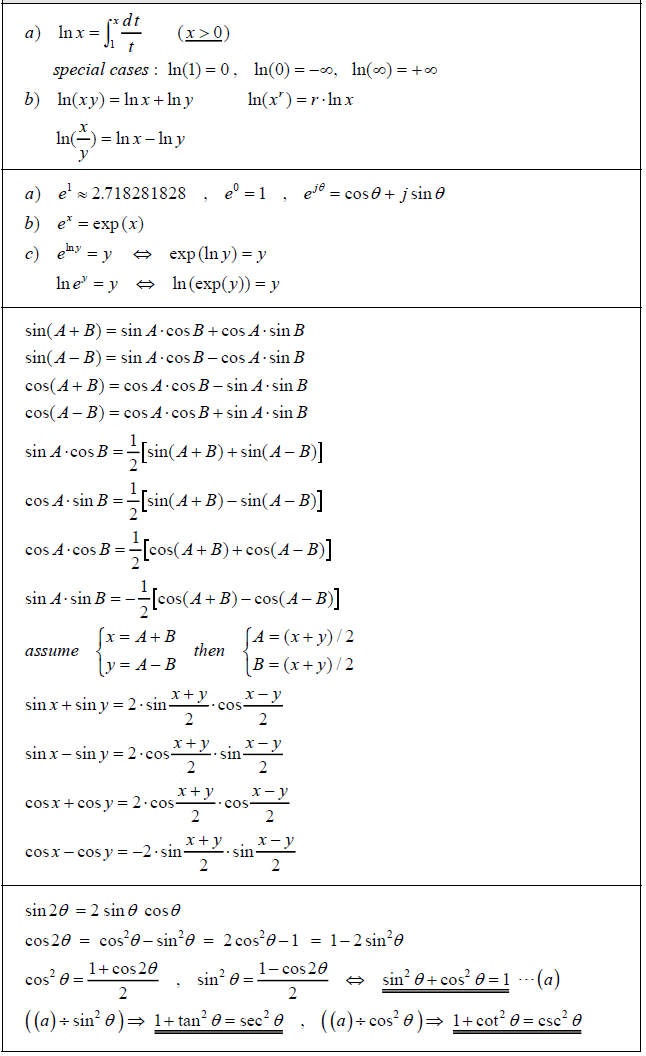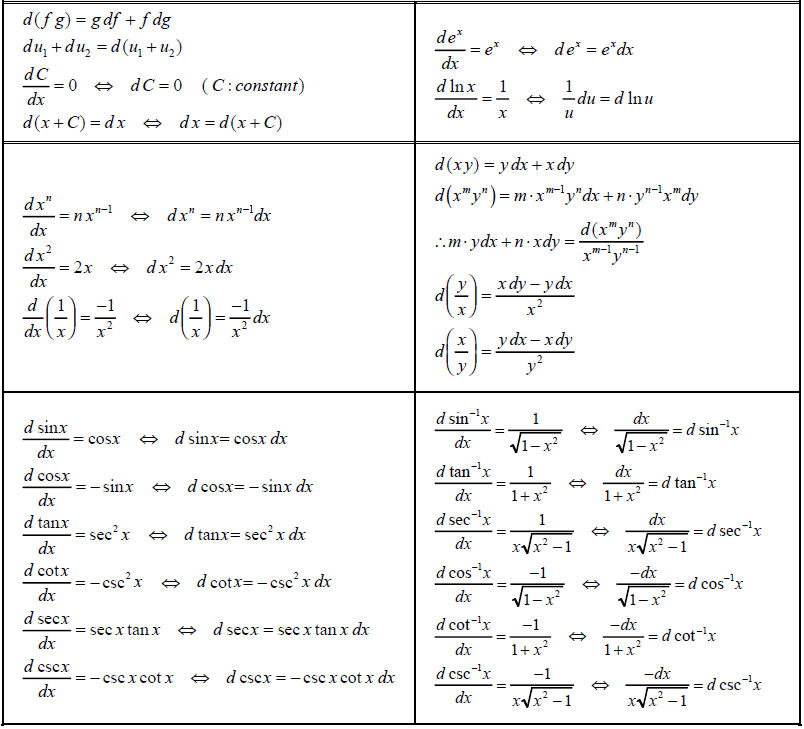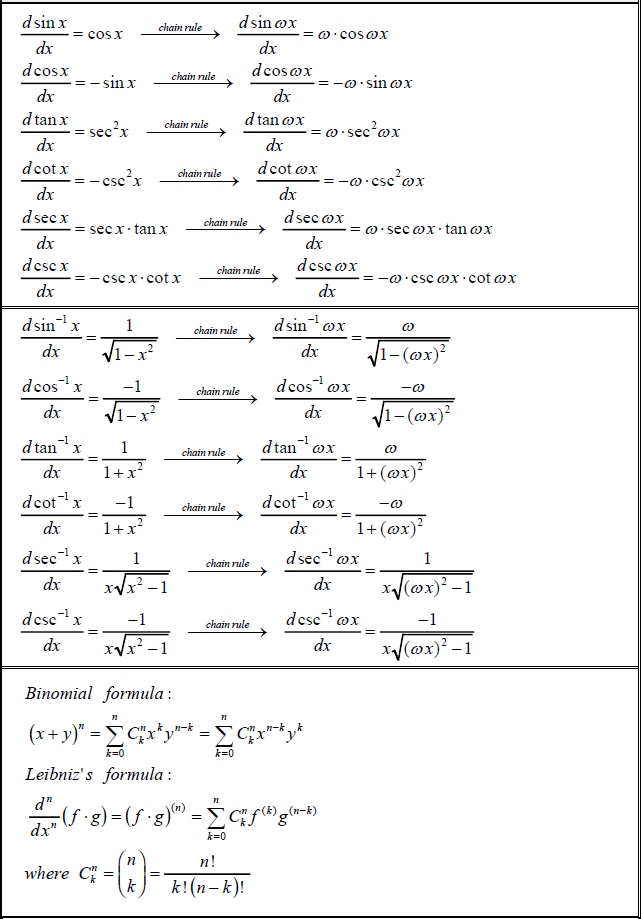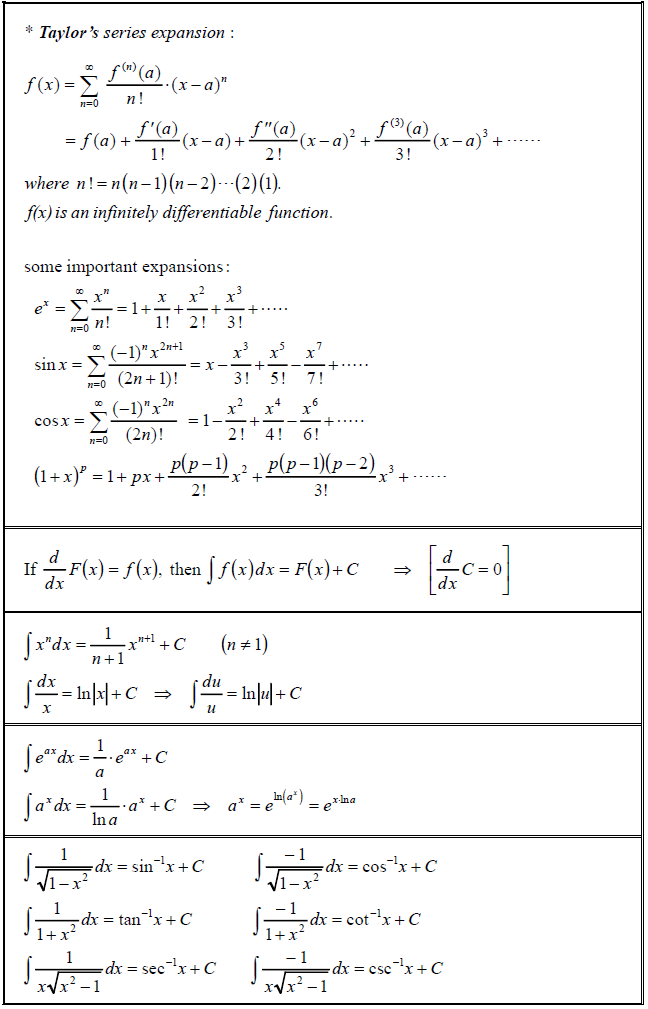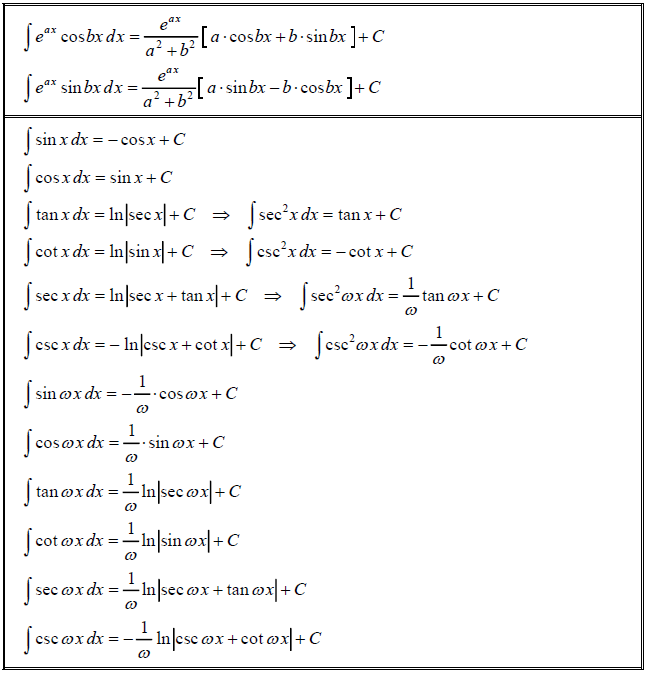### Series Expansion

Complex exponential formula
$\sin(x) = \displaystyle \frac{e^{ix}-e^{-ix}}{2i}$
$\cos(x) = \displaystyle \frac{e^{ix}+e^{-ix}}{2}$
hyperbolic function: $\sinh(x) = -i \sin(ix) \; \cdots$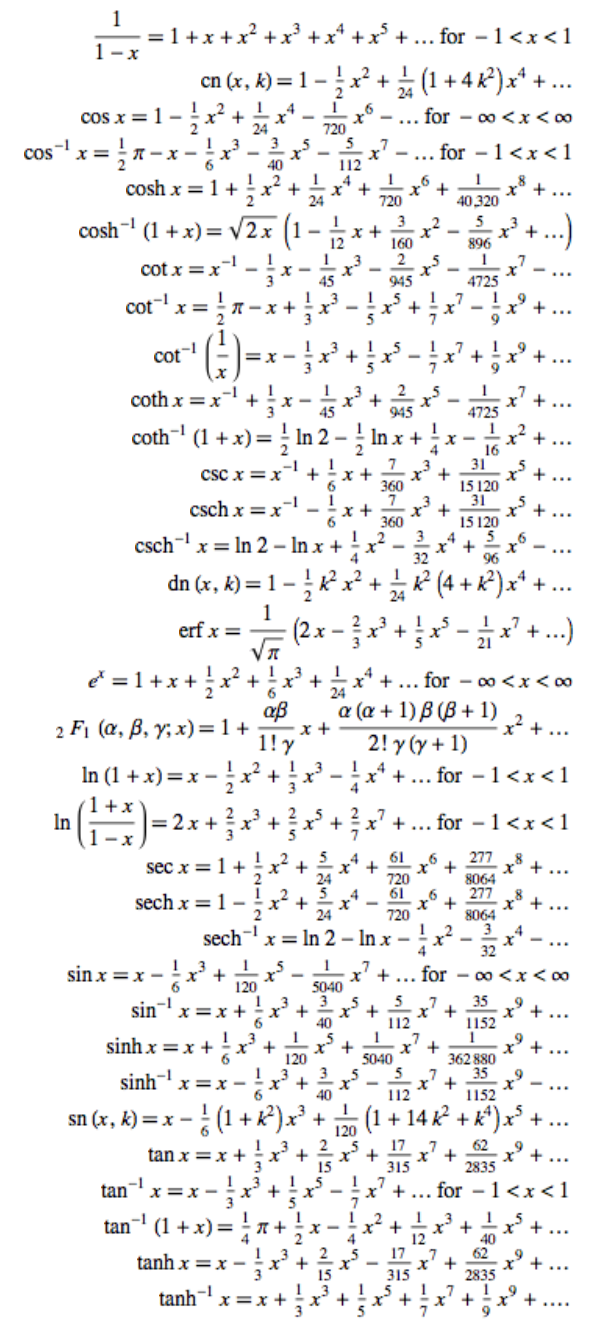Support